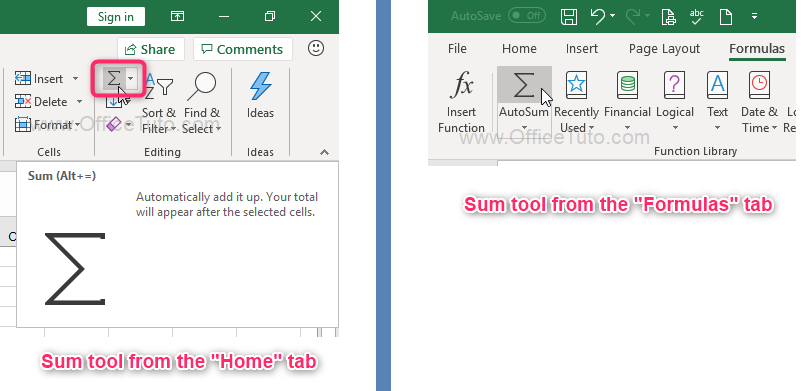Excel Sum function

From the time when formulas and functions feature was developed for Microsoft Excel, SUM is probably the most used function. This clearly signifies the utility of addition of numbers in our daily life.

In this article, we will present the Excel SUM function in detail.

– Introduction

To sum in Excel, you can use the Sum tool from the ribbon (see the screenshot below) or, as any other Excel function, type it manually in a formula.

[ For more insight into Excel formulas, you can see the Excel formulas tutorial ].These 2 ways of calculating a sum in Excel (through the sum tool and manually) can be subdivided into 4 comparable methods:

– 1st method: Select first

• Select the range of data you want to sum.
• Click on the AutoSum tool: As shown in the screenshot above, go to the “Home” tab of the ribbon and in the “Editing” group click on the “AutoSum” tool; or go to the “Formulas” tab and in the “Function Library” click on the “AutoSum” tool .
• Excel automatically calculates the total of the data you selected and puts the result below them if it is a column or on their right if it is a row.
• Press Enter.

– 3rd method: Manually type the Sum formula

In this method, we will manually enter the Sum function in a formula [ If you’re new to Excel formulas, take a look at the Excel formulas tutorial ].

– 4th method: Keyboard shortcut

• Double-click on the result cell to place the cursor in it.
• Use the keyboard shortcut Alt+= (hold down the Alt key and press the = key of the keyboard).
• Excel automatically searches for data around this cell result and selects them. If it is the data range you want to calculate, accept it by pressing Enter; otherwise, select the correct range and then press Enter.

That’s it.

This tutorial was a quick overview of how to sum in Excel using the AutoSum tool and the SUM function; I hope you’ll find it useful.

One Reply to “Excel Sum function”

1.ปั้มไลค์ says:

Like!! I blog quite often and I genuinely thank you for your information. The article has truly peaked my interest.